Students can download Maths Chapter 3 Algebra Ex 3.1 Questions and Answers, Notes, Samacheer Kalvi 10th Maths Guide Pdf helps you to revise the complete Tamilnadu State Board New Syllabus, helps students complete homework assignments and to score high marks in board exams.

## Tamilnadu Samacheer Kalvi 10th Maths Solutions Chapter 3 Algebra Ex 3.1

Question 1.
Solve the following system of linear equations in three variables
(i) x + y + z = 5
2x – y + z = 9
x – 2y + 3z = 16
x + y + z = 5 ….(1)
2x – y + z = 9 ….(2)
x – 2y + 3z = 16 ….(3)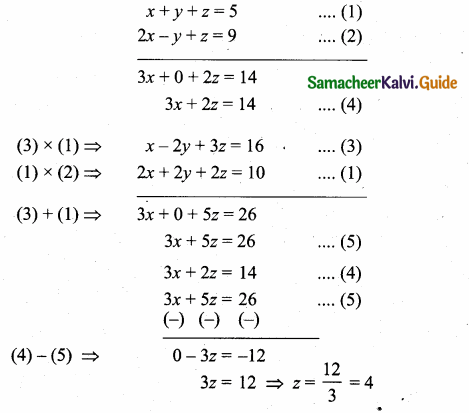Substituting z = 4 (4)
3x + 2(-4) = 14
3x – 8 = 14
3x = 14 – 8
3x = 6
x = $$\frac { 6 }{ 3 }$$ = 2Substituting x = 2 and z = 4 in (1)
2 + y + 4 = 5
y + 6 = 5
y = 5 – 6
= -1
∴ The value of x = 2, y = -1 and z = 4

(ii) $$\frac { 1 }{ x }$$ – $$\frac { 2 }{ y }$$ + 4 = 0, $$\frac { 1 }{ y }$$ – $$\frac { 1 }{ z }$$ + 1 = 0, $$\frac { 2 }{ z }$$ + $$\frac { 3 }{ x }$$ = 14
Let $$\frac { 1 }{ x }$$ = p, $$\frac { 1 }{ y }$$ = q and $$\frac { 1 }{ z }$$ = r
p – 2q + 4 = 0
p – 2q = -4 ……(1)
q – r + 1 = 0
q – r = -1 ……(2)
3p + 2r = 14 ……(3)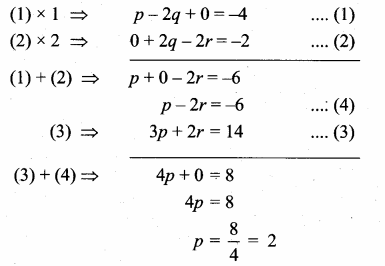Substituting the value of p = 2 in (1)
2 – 2q = -4
-2q = – 4 – 2
-2q = -6
q = $$\frac { 6 }{ 2 }$$ = 3
Substituting the value of q = 3 in (2)
3 – r = 1
– r = – 1 – 3
r = 4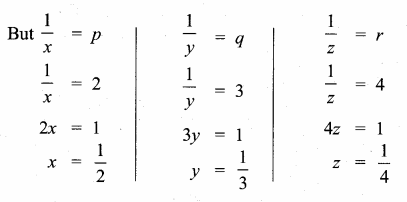The value of x = $$\frac { 1 }{ 2 }$$, y = $$\frac { 1 }{ 3 }$$ and z = $$\frac { 1 }{ 4 }$$(iii) x + 20 = $$\frac { 3y }{ 2 }$$ + 10 = 2z + 5 = 110 – (y + z)
x + 20 = $$\frac { 3y }{ 2 }$$ + 10
Multiply by 2
2x + 40 = 3y + 20
2x – 3y = -40 + 20
2x – 3y = -20 ……(1)

$$\frac { 3y }{ 2 }$$ + 10 = 2z + 5
Multiply by 2
3y + 20 = 4z + 10
3y – 4z = 10 – 20
3y – 4z = -10 ……(2)

2z + 5 = 110 – (y + z)
2z + 5 = 110 – y – z
y + 3z = 110 – 5
y + 3z = 105 ….(3)Substitute the value of z = 25 in (2)
3y – 4(25) = -10
3y – 100 = – 10
3y = – 10 + 100
3y = 90
y = $$\frac { 90 }{ 3 }$$ = 30
∴ The value of x = 35, y = 30 and z = 25

Substitute the value of y = 30 in (1)
2x – 3(30) = -20
2x – 90 = -20
2x = -20 + 90
2x = 70
x = $$\frac { 70 }{ 2 }$$ = 35Question 2.
Discuss the nature of solutions of the following system of equations
(i) x + 2y – z = 6, – 3x – 2y + 5z = -12 , x – 2z = 3
x + 2y – z = 6 …..(1)
-3x – 2y + 5z = -12 …..(2)
x – 2z = 3 ……(3)
Adding (1) and (2) we get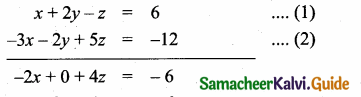Adding (3) and (4) we get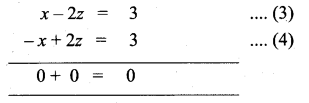The above statement tells us that the system has an infinite number of solutions.

(ii) 2y + z = 3(- x + 1) ,-x + 3y – z = -4, 3x + 2y + z = –$$\frac { 1 }{ 2 }$$
2y + z = 3 (- x + 1)
2y + z = -3x + 3 ……(1)
3x + 2y + z = –$$\frac { 1 }{ 2 }$$

-x + 3y – z = – 4
x – 3y + z = 4 …..(2)

3x + 2y + z = – $$\frac { 1 }{ 2 }$$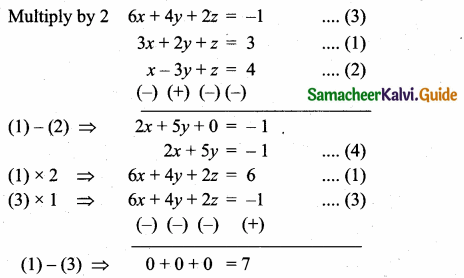Hence we arrive at a contradiction as 0 ≠ 7
This means that the system is inconsistent and has no solution.(iii) $$\frac { y+z }{ 4 }$$ = $$\frac { z+x }{ 3 }$$ = $$\frac { x+y }{ 2 }$$, x + y + z = 27
$$\frac { y+z }{ 4 }$$ = $$\frac { z+x }{ 3 }$$
3y + 3z = 4z + 4x
-4x + 3y + 3z – 4z = 0
-4x + 3y – z = 0
4x – 3y + z = 0 ………(1)

$$\frac { z+x }{ 3 }$$ = $$\frac { x+y }{ 2 }$$
2z + 2x = 3x + 3y
-3x + 2x – 3y + 2z = 0
-x – 3y + 2z = 0
x + 3y – 2z = 0 ………(2)Substituting the value of x in (5)
6 + 5z = 81
5z = 81 – 6
5z = 75
z = $$\frac { 75 }{ 5 }$$ = 15
Substituting the value of x = 3
and z = 15 in (3)
3 + y + 15 = 27
y + 18 = 27
y = 27 – 18
= 9
The value of x = 3, y = 9 and z = 15
This system of equations have unique solution.Question 3.
Vani, her father and her grand father have an average age of 53. One-half of her grand father’s age plus one-third of her father’s age plus one fourth of Vani’s age is 65. If 4 years ago Vani’s grandfather was four times as old as Vani then how old are they all now?
Let the age of Vani be”x” years
Vani father age = “y” years
Vani grand father = “z” years
By the given first condition.
$$\frac { x+y+z }{ 3 }$$ = 53
x + y + z = 159 ….(1)
By the given 2nd Condition.
$$\frac { 1 }{ 2 }$$ z + $$\frac { 1 }{ 3 }$$y + $$\frac { 1 }{ 4 }$$x = 65
Multiply by 12
6z + 4y + 3x = 780
3x + 4y + 6z = 780 ….(2)
By the given 3rd condition
z – 4 = 4 (x – 4) ⇒ z – 4 = 4x – 16
– 4x + z = – 16 + 4
4x – z = 12 ……(3)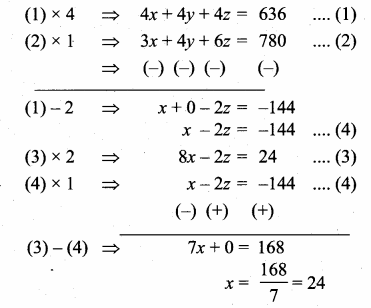Vani age = 24 years
Vani’s father age = 51 years
Vani grand father age = 84 years
Substitute the value of x = 24 in (3)
4 (24) – z = 12
96 – z = 12
-z = 12 – 96
z = 84
Substitute the value of
x = 24 and z = 84 in (1)
24 + y + 84 = 159
y + 108 = 159
y = 159 – 108
= 51Question 4.
The sum of the digits of a three-digit number is 11. If the digits are reversed, the new number is 46 more than five times the old number. If the hundreds digit plus twice the tens digit is equal to the units digit, then find the original three digit number ?
Let the hundreds digit be x and the tens digit be ”y” and the unit digit be “z”
∴ The number is 100x + 10y + z
If the digits are reversed the new number is 100z + 10y + x
By the given first condition
x + y + z = 11 ….(1)
By the given second condition
100z + 10y + x = 5 (100x + 10y + z) + 46
= 500x + 50y + 5z + 46
x – 500x + 10y – 50y + 100z – 5z = 46
– 499x – 40y + 95z = 46
499x + 40y – 95z = -46 ….(2)
By the given third condition
x + 2y = z
x + 2y – z = 0 ….(3)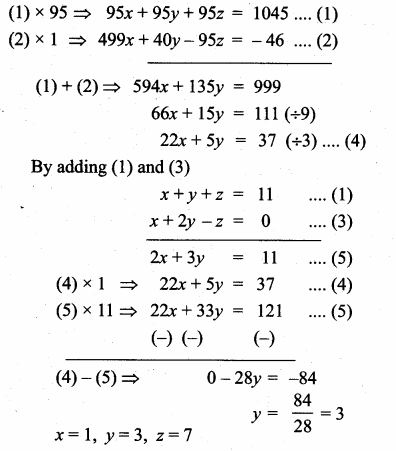∴ The number is 137
Subtituting the value of y = 3 in (5)
2x + 3(3) = 11
2x = 11 – 9
2x = 2
x = $$\frac { 2 }{ 2 }$$ = 1
Subtituting the value of x = 1, y = 3 in (1)
1 + 3 + z = 11
z = 11 – 4
= 7Question 5.
There are 12 pieces of five, ten and twenty rupee currencies whose total value is ₹105. But when first 2 sorts are interchanged in their numbers its value will be increased by ₹20. Find the number of currencies in each sort.
Let the number of ₹5 currencies be “x”
Let the number of ₹10 currencies be “y”
and the number of ₹20 currencies be “z”
By the given first condition
x + y + z = 12 ………(1)
By the given second condition
5x + 10y + 20z = 105
x + 2y + 4z = 21 (÷5) ……….(2)
By the given third condition
10x + 5y + 20z = 105 + 20
10x + 5y + 20z = 125
2x + y + 4z = 25 ………..(3)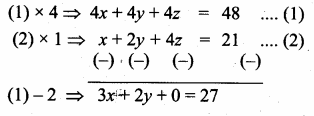Substituting the value of x = 7 in (5)
7 – y = 4 ⇒ -y = 4 – 7
-y = -3 ⇒ y = 3
Substituting the value of x = 7, y = 3 in …. (1)
7 + 3 + z = 12
z = 12 – 10 = 2
x = 7, y = 3, z = 2
Number of currencies in ₹ 5 = 7
Number of currencies in ₹ 10 = 3
Number of currencies in ₹ 20 = 2